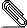# Re: [Haskell-cafe] Re: powerSet = filterM (const [True, False]) ... is this obfuscated haskell?

```2009/7/17 Gleb Alexeyev <gleb.alex...@gmail.com>:
```
```On Jul 17, 2009 1:40pm, Thomas Hartman wrote:
```
`my question to all 3 (so far) respondants is, how does your`
```
explanation explain that the result is the power set?

```
```
Because powerset(s) = 2^s?

I was going to make some nice code but I ended up with this monster :D

{-# LANGUAGE ScopedTypeVariables #-}

-- a more generic "if"
gif p t f
| p == maxBound = t
| otherwise     = f

-- this is filterM, but with the generic if
collect _ [] = return []
collect p (x:xs) = do
flg <- p x
ys <- collect p xs
return (gif flg (x:ys) ys) -- just changed if -> gif

-- list exponentiation -- first parameter is fake, just to get an 'a'
expSet :: forall a b. (Bounded a, Enum a, Eq a) => a -> [b] -> [[b]]
expSet _a = collect (\_-> values :: [a])

values :: (Bounded a, Enum a) => [a]
values = enumFromTo minBound maxBound

data Trool = Un | Deux | Trois deriving (Bounded, Enum, Eq, Show)
trool = undefined :: Trool
bool = undefined :: Bool

powerset = expSet bool

I feel dirty :P```signature.asc
Description: OpenPGP digital signature

```_______________________________________________